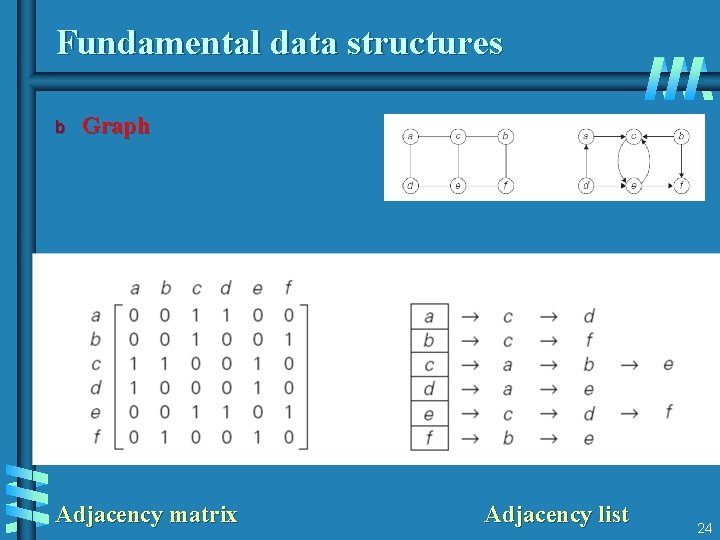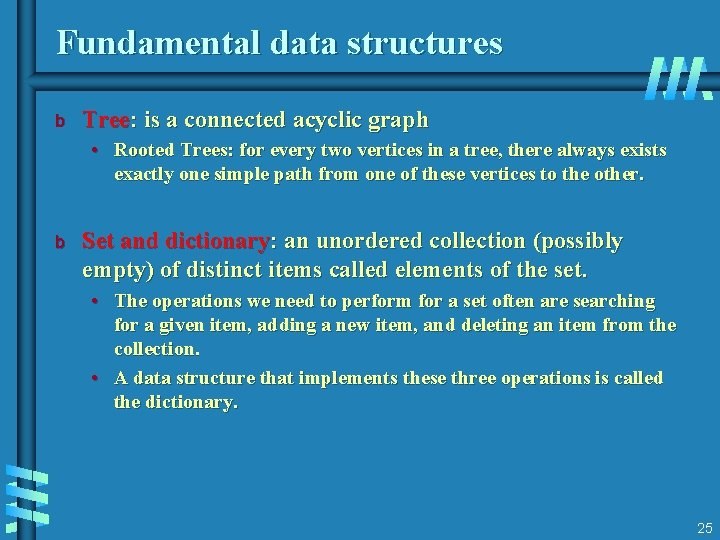# What is an algorithm An algorithm is a

• Slides: 25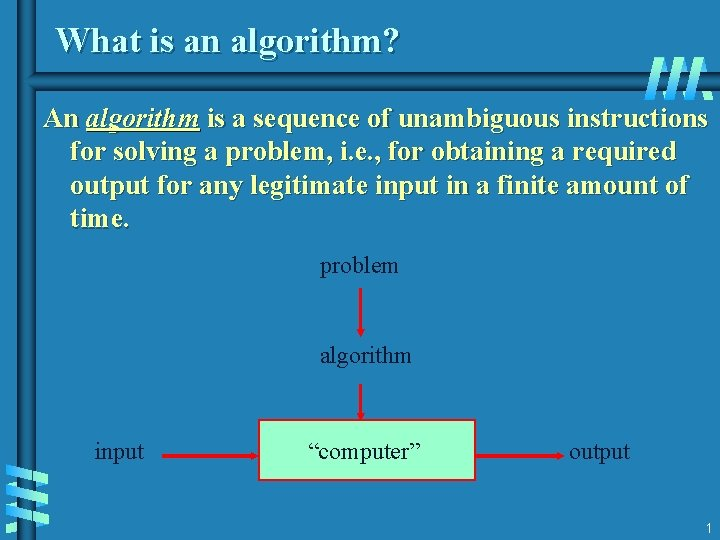What is an algorithm? An algorithm is a sequence of unambiguous instructions for solving a problem, i. e. , for obtaining a required output for any legitimate input in a finite amount of time. problem algorithm input “computer” output 1What is an algorithm? • is any well-defined computational procedure that takes some value, or set of values, as input and produces some value, or set of values, as output. • is thus a sequence of computational steps that transform the input into the output. • is a tool for solving a well - specified computational problem. • Any special method of solving a certain kind of problem (Webster Dictionary( 2What is a problem? b Definition • A mapping/relation between a set of input instances (domain) and an output set (range) b Problem Specification • Specify what a typical input instance is • Specify what the output should be in terms of the input instance b Example: Sorting • Input: A sequence of N numbers a 1…an • Output: the permutation (reordering) of the input sequence such that a 1 a 2 … an. 3Types of Problems b Search: find X in the input satisfying property Y b Structuring: Transform input X to satisfy property Y b Construction: Build X satisfying Y b Optimization: Find the best X satisfying property Y b Decision: Does X satisfy Y? 4What do we analyze about algorithms b Correctness • Does the input/output relation match algorithm requirement? b Amount of work done (aka complexity ( • Basic operations to do task b Amount of space used • Memory used 5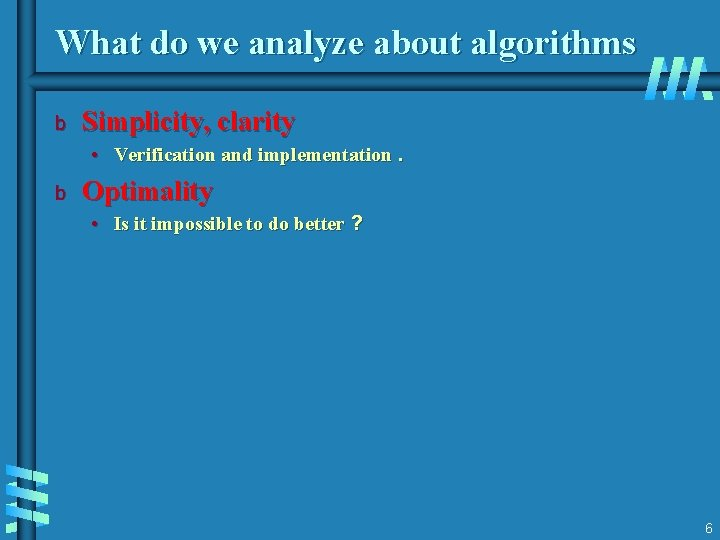What do we analyze about algorithms b Simplicity, clarity • Verification and implementation. b Optimality • Is it impossible to do better ? 6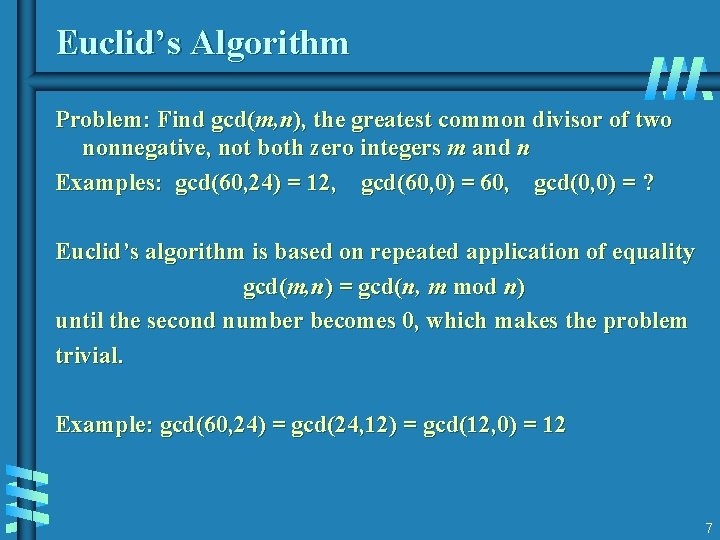Euclid’s Algorithm Problem: Find gcd(m, n), the greatest common divisor of two nonnegative, not both zero integers m and n Examples: gcd(60, 24) = 12, gcd(60, 0) = 60, gcd(0, 0) = ? Euclid’s algorithm is based on repeated application of equality gcd(m, n) = gcd(n, m mod n) until the second number becomes 0, which makes the problem trivial. Example: gcd(60, 24) = gcd(24, 12) = gcd(12, 0) = 12 7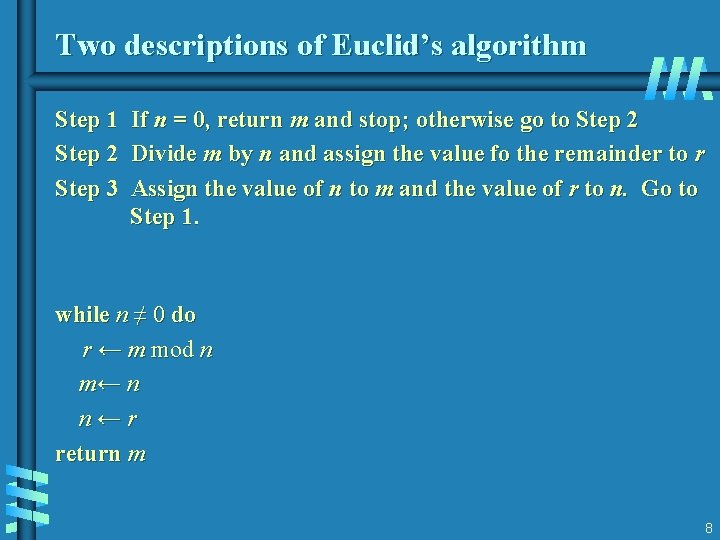Two descriptions of Euclid’s algorithm Step 1 Step 2 Step 3 If n = 0, return m and stop; otherwise go to Step 2 Divide m by n and assign the value fo the remainder to r Assign the value of n to m and the value of r to n. Go to Step 1. while n ≠ 0 do r ← m mod n m← n n←r return m 8Other methods for computing gcd(m, n) Consecutive integer checking algorithm Step 1 Assign the value of min{m, n} to t Step 2 Divide m by t. If the remainder is 0, go to Step 3; otherwise, go to Step 4 Step 3 Divide n by t. If the remainder is 0, return t and stop; otherwise, go to Step 4 Decrease t by 1 and go to Step 2 9Other methods for gcd(m, n) [cont. ] Middle-school procedure Step 1 Step 2 Step 3 Step 4 Find the prime factorization of m Find the prime factorization of n Find all the common prime factors Compute the product of all the common prime factors and return it as gcd(m, n) Is this an algorithm? 10Sieve of Eratosthenes Input: Integer n ≥ 2 Output: List of primes less than or equal to n for p ← 2 to n do A[p] ← p for p ← 2 to n do if A[p] 0 //p hasn’t been previously eliminated from the list j ← p* p while j ≤ n do A[j] ← 0 //mark element as eliminated j←j+p Example: 2 3 4 5 6 7 8 9 10 11 12 13 14 15 16 17 18 19 20 11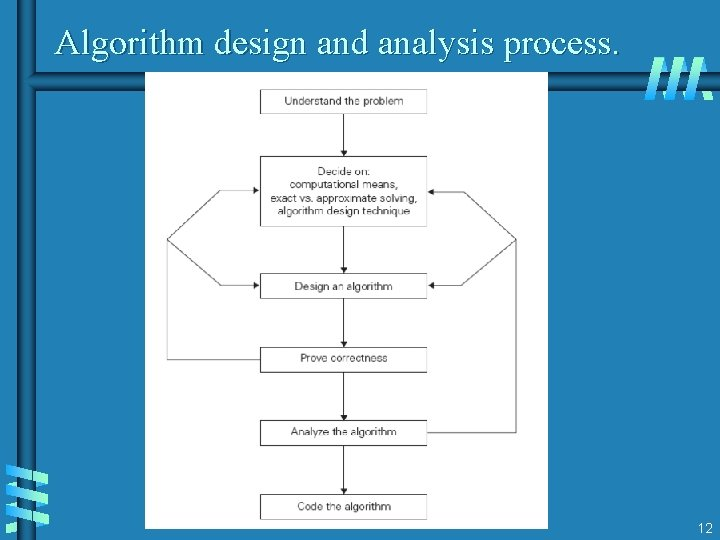Algorithm design and analysis process. 12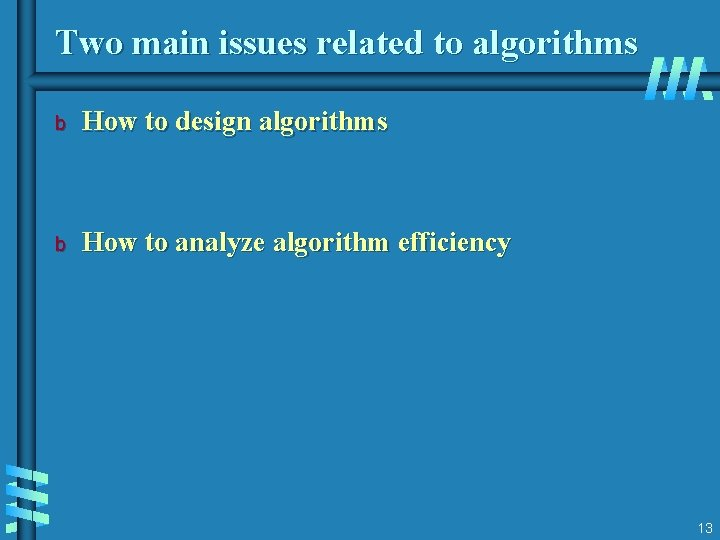Two main issues related to algorithms b How to design algorithms b How to analyze algorithm efficiency 13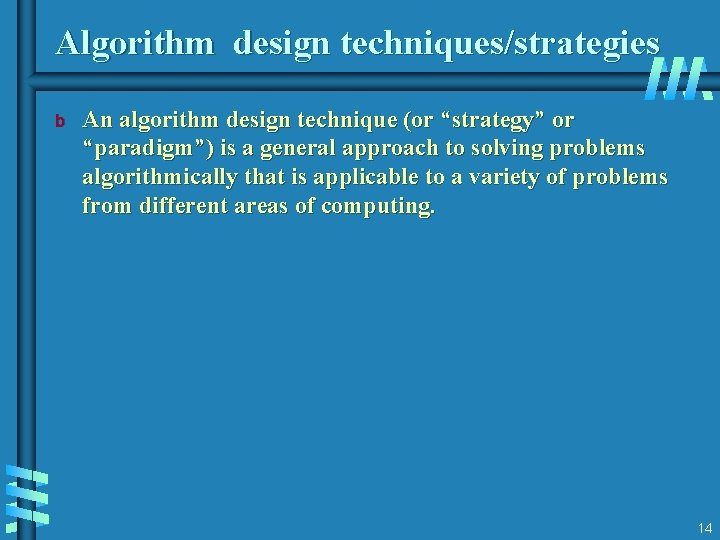Algorithm design techniques/strategies b An algorithm design technique (or “strategy” or “paradigm”) is a general approach to solving problems algorithmically that is applicable to a variety of problems from different areas of computing. 14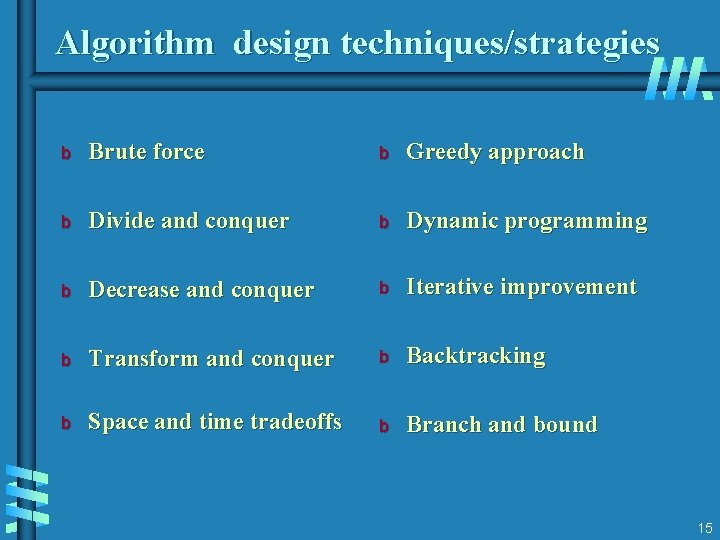Algorithm design techniques/strategies b Brute force b Greedy approach b Divide and conquer b Dynamic programming b Decrease and conquer b Iterative improvement b Transform and conquer b Backtracking b Space and time tradeoffs b Branch and bound 15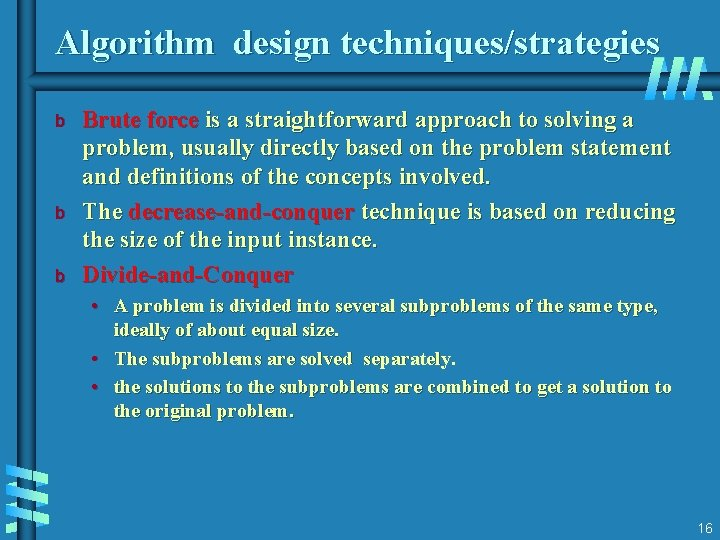Algorithm design techniques/strategies b b b Brute force is a straightforward approach to solving a problem, usually directly based on the problem statement and definitions of the concepts involved. The decrease-and-conquer technique is based on reducing the size of the input instance. Divide-and-Conquer • A problem is divided into several subproblems of the same type, ideally of about equal size. • The subproblems are solved separately. • the solutions to the subproblems are combined to get a solution to the original problem. 16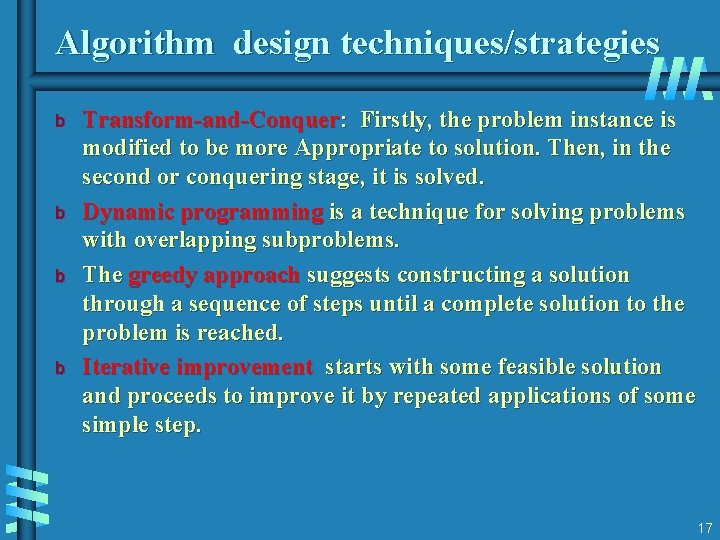Algorithm design techniques/strategies b b Transform-and-Conquer: Firstly, the problem instance is modified to be more Appropriate to solution. Then, in the second or conquering stage, it is solved. Dynamic programming is a technique for solving problems with overlapping subproblems. The greedy approach suggests constructing a solution through a sequence of steps until a complete solution to the problem is reached. Iterative improvement starts with some feasible solution and proceeds to improve it by repeated applications of some simple step. 17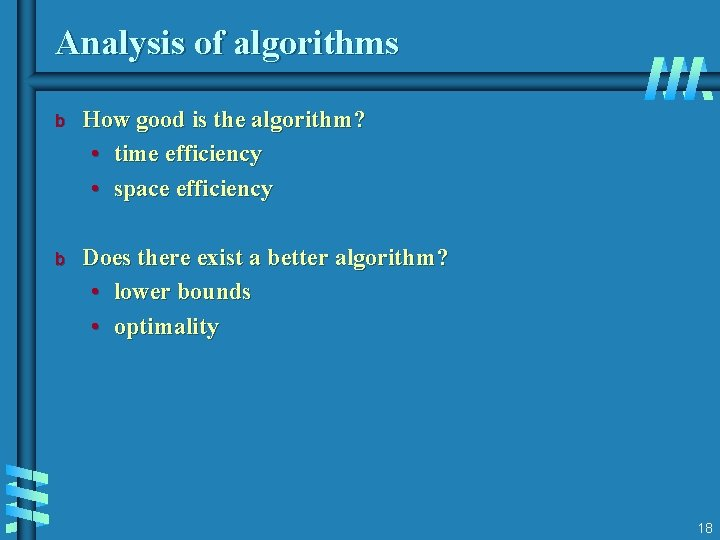Analysis of algorithms b How good is the algorithm? • time efficiency • space efficiency b Does there exist a better algorithm? • lower bounds • optimality 18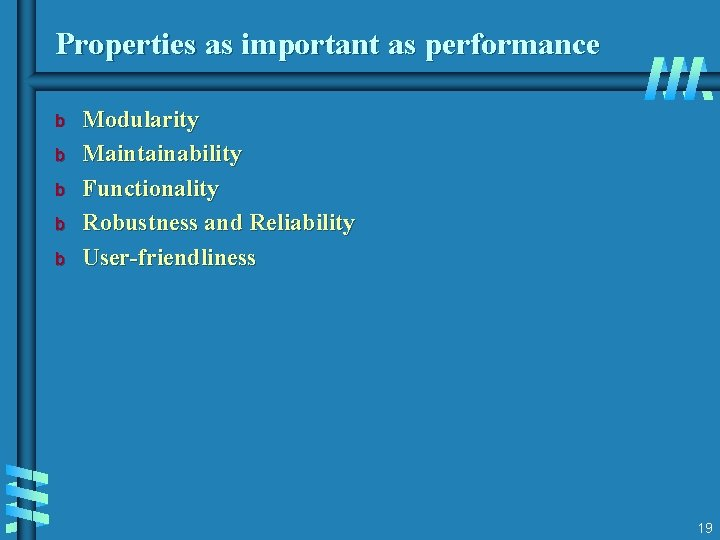Properties as important as performance b b b Modularity Maintainability Functionality Robustness and Reliability User-friendliness 19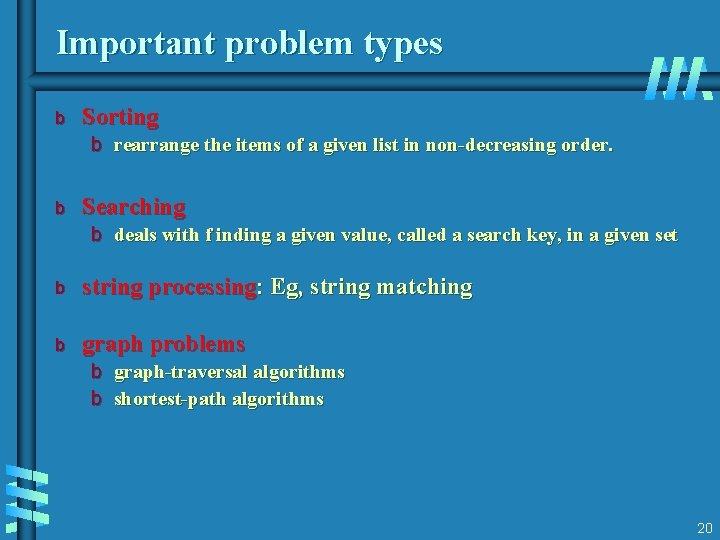Important problem types b Sorting b rearrange the items of a given list in non-decreasing order. b Searching b deals with f inding a given value, called a search key, in a given set b string processing: Eg, string matching b graph problems b graph-traversal algorithms b shortest-path algorithms 20Important problem types b Combinatorial problems: find a combinatorial object— such as a permutation, a combination, or a subset—that satisfies certain constraints. b Geometric problems: deal with geometric objects such as points, lines, and polygons. b Numerical problems: involve mathematical objects of continuous nature: b b solving equations and systems of equations, computing definite integrals, evaluating functions, and …. . so on. 21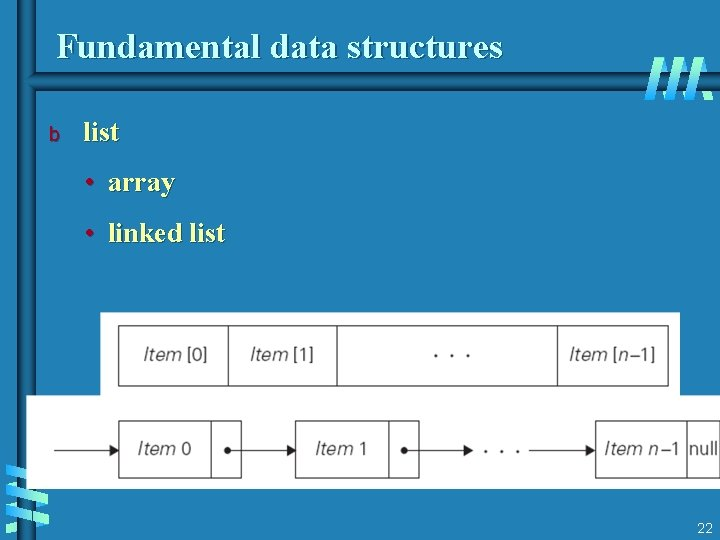Fundamental data structures b list • array • linked list 22Fundamental data structures b stack b queue b priority queue 23